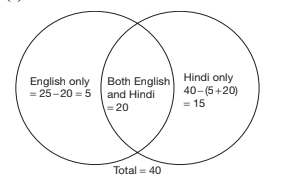# Mathematical Aptitude - Venn Diagram

>>>>>>>>Venn Diagram

Study the diagram given below and answer each of the following questions.• A

5• B

8• C

25• D

16Study the diagram given below and answer each of the following questions.• A

25• B

17• C

5• D

10Study the diagram given below and answer each of the following questions.• A

10• B

17• C

30• D

15• A

47• B

43• C

53• D

58• Option : C
• Explanation : Let us draw a Venn diagram with the information given in the question.Numbers divisible by 3 (3, 6, 9 and so on) = 33
Numbers divisible by 5 (5, 10, 15 and so on) = 20
Numbers which are divisible by both 3 and 5 (15, 30, 45, 60, 75 and 90) = 6
Numbers divisible by 3 or 5 or both 3 and 5 = 27 + 6 + 14 = 47 (alternatively, 33 + 20 − 6 = 47)
Numbers neither divisible by 3 nor by 5 = 100 − 47 = 53

• A

15• B

20• C

25• D

30• Option : A
• Explanation :## Description

• Venn Diagrams Questions can be used to give quizzes by any candidate who is preparing for UGC NET Paper 1
• This Venn Diagrams Questions section will help you test your analytical skills in a tricky method, thereby giving you an edge over other students
• All candidates who have to appear for the Kendriya Vidyalaya Entrance exam can also refer to this mcq section.
• You can also get access to the Venn Diagrams MCQ ebook.
• Venn Diagrams Questions can be used in the preparation of JRF, CSIR, and various other exams.